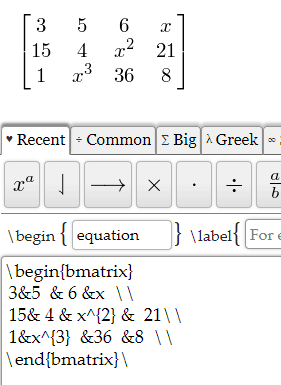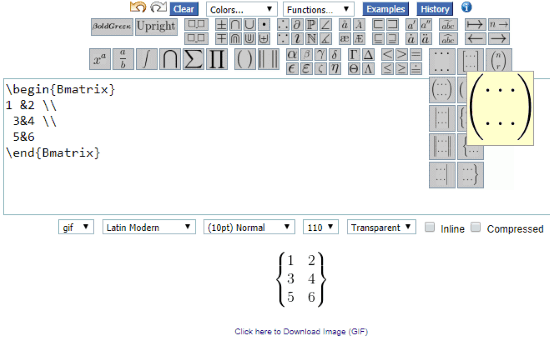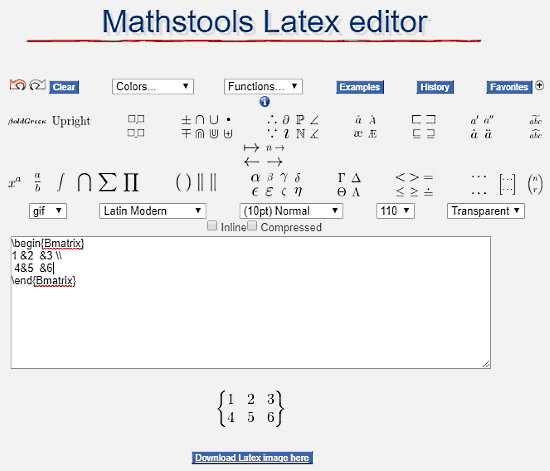Editor Ratings:
User Ratings:
[Total: 1 Average: 2]

LaTex is a great medium to write mathematical or scientific equations. There are several online LaTex editors, and using all of them one can create matrices of custom dimension. When all of them can create matrix, then why create this separate list of LaTex Matrix Generator websites? Well, some of those editors are plain editors, where you will have to write LaTex code yourself. While some of them only provide LaTex matrix equation for 2×2 matrix, and need modification.

So, what’s so special about these LaTex matrix generator websites which I have mentioned in this list? These tools let you enter the matrix dimension before you create a LaTex matrix. Moreover, you do not even have to play around with LaTex matrix equation. All you need to do is enter values in the equation. After creating matrix, you will be able to download it as image on your PC.

Note: All of the mentioned editors are full fledged LaTex editors, and the discussions will only be limited to matrix generator capabilities. If you are looking just for Online Latex Editor, click here.

## Formula SheetIn my opinion, Formula Sheet is the best Online LaTex matrix generator out there. How? It provides the easiest solution to create matrix in a jiffy. Open the LaTex editor, then go to the Matrix tab from the panel at the bottom. Here, you will find various types of matrices. Select one, then enter the matrix dimensions, and click on Create Matrix button.

Now the LaTex matrix equation will be rendered in the box below the panel. This is what the empty LaTex matrix equation for a 3×4 matrix looks like:In this equation template, you have to enter the values before & sign. Its quite obvious how you have to enter the values of matrices according to their position. Let me provide an example for this tool, in case you are not so familiar with LaTex.As you can see, before each & sign, I entered some values, which show up in the matrix above.

To save the rendered matrix, simply right click on the rendered matrix, and click on Save Image as option.

## Wiris EditorWiris Editor is another good and simple LaTex Matrix generator. Along with LaTex, it is also MathML compatible. So, when you create a matrix, both LaTex and MathML codes are displayed.

To begin with, when the editor opens, go to the matrix tab. Now, select the type of matrix you want to create. A pop up comes up as displayed above. Here, you can select the dimension of matrix by clicking. You can also enter matrix dimension manually in the pop up. After that, an empty matrix box comes up. Here, you do not even have to play with the LaTex matrix equation. Simply fill the empty matrix boxes. If you want to enter any LaTex code, you can do that from the LaTex box below.

With this editor, you cannot save the matrix as an image. Then what is this tool doing on number 2? Well, it is very suitable for people who do not know LaTex properly, and need to quickly create a matrix. After that, they can copy the matrix code, and use it in their projects.

## Number Empire: LaTex Equation EditorLaTex Equation Editor from Number Empire is another great tool to create LaTex matrix. You can right away start building matrix as you open the editor. Click on the matrix option at the top right corner of toolbox. From here, select the type of matrix you would like to create. After selecting the matrix, a pop up will appear at top of the website. Here, enter the matrix dimensions separated by a comma. After that, the LaTex matrix equation will appear in the box. Here, you will have to enter matrix components as discussed in Formula Sheet section. When done, click on the Render Equation button. To save the matrix as image, right click on the rendered matrix and select Save image as option.

## CodecogsCodecogs is just like the above mentioned tool. The only difference is that you do not need to click the render button. After selecting matrix and entering dimensions, you need to put values in LaTex equation. The changes will appear in the rendered matrix in real time.

When you select the matrix from the tool panel, the same kind of pop up as above will appear on top of website.

You can save the rendered matrix as PNG, GIF, PDF, SVG, etc. formats.

## Mathstool LaTex EditorMathstool LaTex Editor is another good option to make Latex Matrix online. From the tool panel, select the matrix type. Now, after selecting matrix, a popup will appear to enter matrix dimensions. Again, the matrix equation will appear in the box, which you have to edit to enter matrix values before & sign. The matrix will be updated as you enter values. You can download image of matrix on your PC as well.

### Closing Words

Out of the various online LaTex editors out there, these are the best bets to create LaTex Matrix. These LaTex Matrix Generator websites are not only good at creating matrix but carrying out various other LaTex operations.

 Editor Ratings: User Ratings:[Total: 1 Average: 2] Free/Paid: Free Tags: latex editor